Published: 30 June 2014

# 3-D calibration method and algorithm for freehand image of phased array ultrasonic testing

Yao Rao1
Jia Cili2
Lu Wenhua3
Cheng Minye4
Yao Peng5
1, 2, 3, 4Aeronautic College, Shanghai University of Engineering Science, Shanghai, 201620, China
5School of Economics & Management, Beihua University, Jilin, 132000, China
Corresponding Author:
Yao Rao
Views 103

#### Abstract

Phased array ultrasonic testing (UT) is an advanced technique applying ultrasound wave vibration theory to detect the flaw in tested materials by imaging. In this research, computer 3-D visualization of the flaw through calibrating the ultrasonic phased array image is proposed. 3-D calibration for ultrasonic phased array image is a procedure to calculate the spatial transformation matrix, spatial relationship between the US image plane and the tracker attached to the UT probe. The calibration method depends on a cross-string phantom and the corresponding algorithm. The phantom with a set of crosses guiding the operator quickly to find the scanning plane. The ten string crosses in the scanning plane provide the coordinates and spatial vectors for the calibration algorithm, thus the calibration algorithm can be realized based on the least-squares fitting method of the homologous points matching. Select the points having different distances and angles with the reference point to calculate the matrix and average them as the final result. The results show that the scanning plane positioning time is no more than 5 s. The precision and the accuracy results are the same as that is obtained through the other published methods in the medical 3-D ultrasound image calibration. The results make the 3-D flaw model reconstruction possible in phased array ultrasonic NDT. It will reduce the difficulties in the flaw recognizing and localization.

## 1. Introduction

Ultrasonic testing (UT) is an application technique of the ultrasound wave vibration theory. It is generally used for flaw detection and localization in tested materials. The difficulties on the flaw recognizing and localization are the extremely important problems when the flaw detection result is shown as the wave type or in the 2-D images. That requires specialized experts and sophisticated assessment tools for detecting the damage. Therefore, some researchers have focused on how to make the flaw 3-D display to reduce the difficulties recently. The current theories and methods are based on phased array [1-3], air-coupled  and 3-D laser .

In the last decade, phased array theory was applied to the ultrasound, and promoted the development of ultrasound phased array. The ultrasound phased array was applied to the researches from medical to industry. Ultrasonic phased array testing is investigated and is replacing the conventional ultrasonic testing technique. Their imaging modes range from A-scans to 3-D ultrasound reconstructions. Although ultrasonic phased arrays are potentially ideally suited to 3-D applications in NDT, their use in practice is limited by the complex probe manufacture for 2-D phased array and the bulk and cost of the associated electronic control instrumentation . In this article the flaw 3-D visualization based on the 2-D ultrasound image provided by one-dimensional phased array probe is investigated. The requirements of the method are the ultrasound image calibration procedure containing the phantom design and the calibration algorithm.

The ultrasound image calibration is an obligatory procedure for 3-D flaw reconstruction. In this research the images are obtained by freehand scanning, that is the operators hold the US probe to collect the image sequences, a series of non-parallel and irregular two-dimensional (2-D) ultrasound images. VTK (Visualization ToolKit) is a reconstruction software tookits for regular parallel image sequences. So these 2-D ultrasound images must be transformed into 3-D space firstly, then pixel interpolating to build the volumn data. In these volumn data the regular and parallel image sequences can be extracted. Thus the 3-D reconstruction tookit will be feasible on 3-D reconstruction for the extracted parallel image sequences. The above mathematical transformation procedure for 2-D coordinates of pixels in the US image to the 3-D coordinates is the US image 3-D calibration. The core algorithm is calculating the transformation relationship between the US probe and the tracking device attached to the US probe . The transformation cannot be physically measured, only can be shown by a 4×4 matrix (rotation, translation and scale).

The performance evaluations of the ultrasound image calibration mainly include the phantom design, the operation speed, the calibration precision and the calibration accuracy . From Detmer initially proposed a point target method and iterative least-squares fitting method to find the calibration matrix, the researches on the calibration phantom, the speed, the precision and the accuracy were uninterrupted. In the aspect of the calibration precision improvement, researchers have mainly focused on the optimization of the solid model with special geometric characteristics known (commonly be called phantom) to reduce the errors caused by interaction operations and calibration algorithms. Though the single point calibration method produced good results, it was time-consuming because it required collection of a large number of US images .

So in the last decade, to simplify the interaction and save the operating time, several phantoms and algorithms were researched (Prager in 1998; Blackall in 2000; Pagoulatos and Muratore in 2001; Letotta in 2004; Sangita in 2005; Hsu in 2008). Abeysekera and De in 2011, Melvaer and Lang in 2012 successively investigated their own optimized methods for phantoms and corresponding algorithms. To accelerate the calibration speed, the real-time 3-D US calibration methods were reported (Poon et al., 2005; Hartov et al., 2010; Hsu et al., 2008; Chen et al., 2009-2011).

In our research, we proposed a cross-string phantom to accelerate the calibration speed in the interaction operations procedure. A tracker was fixed on the ultrasound probe, during the scanning on the crosses in the phantom, real-time recording the probe’s position and orientation. More importantly, the different calibration algorithm based on the space vector was researched, which could optimize the least-squares fitting algorithm to calculate the calibration matrix based on the phased array ultrasound image, and maintained the precision and the accuracy results the same as that is obtained through the other published methods in the medical 3-D ultrasound image calibration. The results make the 3-D flaw model reconstruction possible in phased array ultrasonic NDT. It will reduce the difficulties in the flaw recognizing and localization.

## 2. Material and method

### 2.1. Theory of calibration algorithm

Figure 1 is the spatial relationships of the devices of the 3D phased array ultrasonic calibration system. The calibration is a procedure to calculate the spatial transformation relationships between the US probe coordinate system and the tracker coordinate system ${T}_{r←i}$. Current tracking sensors mainly include the magnetic tracker and the optical tracker. We selected the magnetic tracker which was also called magnetic receiver. When select the magnetic tracker, the metal influence of the magnetic field must be considered, because the ultrasonic testing is different from the medical ultrasound, its probe usually is manufactured with metal and it is probably used to test the metal materials.

Finally the image calibration ${T}_{r←i}$ is the matrix:

1
${T}_{r←i}={T}_{t←r}^{-1}\cdot {T}_{p←t}^{-1}\cdot {T}_{p←i}.$

Fig. 1Coordinate systems definition and transformation relationships include receiver transmitter, receiver, phantom and image coordinate systems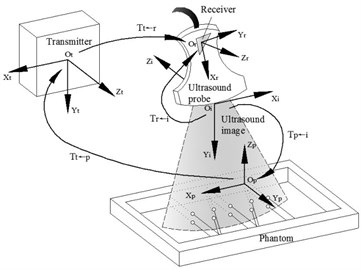### 2.2. Phantom and ${\mathbit{T}}_{\mathbit{p}←\mathbit{t}}$ calibration

In order to obtain ${T}_{r←i}$, ${T}_{p←t}$ must be required. ${T}_{p←t}$ can be obtained by using the probe to scan a phantom with special geometric characteristic points. As is presented in Figure 2, we design a cross-string phantom which is consisted of cross-string, their planar arrays and phantom frame. The cotton strings of the cross-string are 0.3 mm in diameter. Because of their elasticity, they can keep tightened to maintain the string’s position precision both in dry and wet conditions in water. The cotton stings pass through the holes, 1 mm in diameter, on both front and back walls of the phantom frame. There are two layers of cotton strings arrays in the middle of the phantom frame.

Fig. 2Phantom construction and coordinate systems definition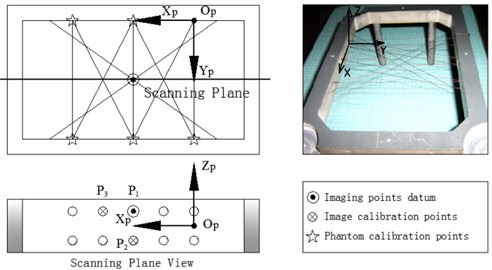The 10 holes in the two opposite sides of the phantom frame which are the endpoints (holes) of the ten vertical strings passing through the phantom are characteristic points for the phantom calibration (Figure 2). They are marked as the stars, double 2 endpoints in the up side and double 3 endpoints in the down side. The phantom calibration is as followings. All coordinates of the marked points in the phantom coordinate system ${P}_{p}$ are given when the phantom is designed. Place the needle attached with the tracker (magnetic receiver) at the characteristic holes on the phantom, through magnetic tracking algorithm ${T}_{t←r}$, the coordinates of these holes in $Ot$ (the magnetic transmitter coordinate system) are be calculated, marked as ${P}_{t}$.

### 2.3. Imaging

Place the US probe upon the cross-strings align with the middle string cross planar arrays (Figure 3), adjust the probe’s orientation and position, to ensure the ultrasound plane aligning with the cross-string layers. Until the 10 middle points are all in the US image, two layers, 5 imaging points in each layer, total 10 imaging points are shown. In this procedure, the strings trend nearby the middle cross arrays are help the probe find the middle cross arrays rapidly. The image origin is defined at the top centre of the sector surface of the US image (Figure 3).

Fig. 3Probe scanning and ultrasound imaging Place the probe upon the middle crosses planar of the strings and ten lightened points in the US image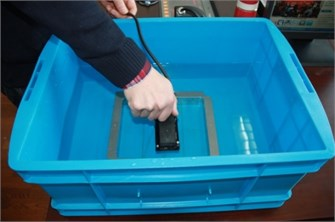a)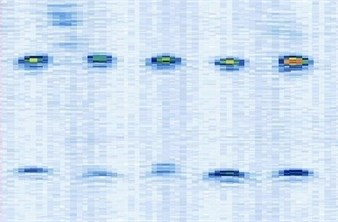b)

### 2.4. Image calibration algorithm

Figure 4 shows the image calibration algorithm. There are ten imaging points in the ultrasound probe scanning image. They are the corresponding images of the cotton strings crosses in the phantom. The algorithm concerns about two points’ coordinates, and they are ${I}_{1}$ and ${I}_{3}$ (Fig. 4(b)). The coordinates of ${P}_{1}$, ${P}_{2}$, ${P}_{3}$, ${d}_{12}$ and ${d}_{23}$ in the phantom are known by design, so the angle $\theta$ between $\stackrel{-}{{P}_{1}{P}_{3}}$ and $\stackrel{-}{{P}_{1}{P}_{2}}$ can be calculated by ${P}_{1}$, ${P}_{2}$ and ${P}_{3}$ (Fig. 4(a)). Then $\theta$ with the distances $\mathrm{}{d}_{12}$ and ${d}_{23}$ provide three parameters for calculating the image point ${I}_{2}$ and ${I}_{3}$.

Fig. 4Image calibration algorithm: a) Homologous points and matching parameters in phantom; b) Homologous points in image and algorithm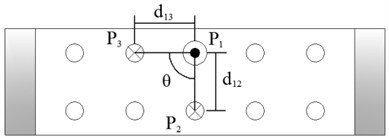a)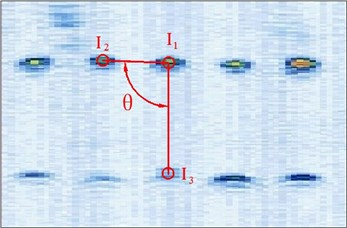b)

The calibration procedure is as followings:

(1) Manual mark ${I}_{1}$. ${I}_{1}$ is the middle imaging point of the middle cross ${P}_{1}$ of the up layer of the ten crosses in the phantom. In this algorithm ${I}_{1}$ is elected as the reference point, because it is nearby the centre line of the sector region in the US image. As the reference of the other points, the distances between other points and it should be calculated. ${I}_{1}$ in the image and ${R}_{1}$ in the receiver are the two homologous points. Where ${R}_{1}$ in the receiver in the receiver is calculated by ${R}_{1}={T}_{r←t}\cdot {T}_{t←p}\cdot {P}_{1}$. Mark ten times repeatedly and average them as the result of the reference coordinate.

(2) Manual mark and calculate ${I}_{2}$. ${I}_{2}$ is a point near the reference point ${P}_{2}$ of the layer below of the ten points sequence in the scanning image. The algorithm requires to mark the highlight point below the reference point ${I}_{1}$, thus the direction of the vector $\stackrel{-}{{I}_{1}{I}_{2}}$ is obtained. Then calculating the distance from ${I}_{1}$ to ${I}_{2}$ according to ${d}_{12}$ (be converted to pixel). Which is determined by the crosses distance in the phantom. ${I}_{2}$ in the image and ${R}_{2}$ in receiver are the two homologous points. Where ${R}_{2}$ in the receiver is calculated by ${R}_{2}={T}_{r←t}\cdot {T}_{t←p}\cdot {P}_{2}$.

(3) Calculate ${I}_{3}$. ${I}_{3}$ is a point near the left side of the reference point ${P}_{3}$ along the vector $\stackrel{-}{{I}_{1}{I}_{3}}$. The direction of $\stackrel{-}{{I}_{1}{I}_{3}}$ is calculated by $\stackrel{-}{{I}_{1}{I}_{2}}$. And the distance from ${I}_{1}$ to ${I}_{3}$ can be calculated according to ${d}_{13}$ (be converted to pixel). Which is determined by the crosses distance in the phantom. ${I}_{3}$ in the image and ${R}_{3}$ in the receiver are the homologous points. Where ${R}_{3}$ in the receiver is ${R}_{3}={T}_{r←t}\cdot {T}_{t←p}\cdot {P}_{3}$.

(4) Apply the least-square fitting to ${P}_{r}\left(R\right)$ and ${P}_{i}\left(I\right)$:

2
$\underset{R,p}{\mathrm{m}\mathrm{i}\mathrm{n}}{\sum ‖{P}_{r}-\left(sR{P}_{i}+p\right)‖}^{2},$

where $s$ is the coefficients of the image (scales, mm/pixel). Calculate the transformation matrix ${T}_{r←i}=\left(R,p\right)$ ($R$: rotation matrix, $p$: translation vector) by the theory of finding the minimized distance between the homologous points ${P}_{r}$ and ${P}_{i}$ . ${T}_{r←i}$ is a 4×4 matrix (rotation, translation and scale) shown as Eq. (3). Its first three columns form $R$ and the fourth column forms $p$.

3
${T}_{r←i}=\left[\begin{array}{cccc}{P}_{11}& {P}_{12}& {P}_{13}& {P}_{14}\\ {P}_{21}& {P}_{22}& {P}_{23}& {P}_{24}\\ {P}_{31}& {P}_{32}& {P}_{33}& {P}_{34}\\ 0& 0& 0& 1\end{array}\right].$

(5) Select different distances points from the reference ${I}_{1}$ as the algorithm ${I}_{2}$ and ${I}_{3}$ like Figure 6, repeat (2)-(4). Then average the calibration matrixes as the ultimate result ${T}_{r←i}$.

The calibration matrix can be calculated from a single US image. However, due to the variations when the probe is placed upon the phantom to find the scanning plane with freehand scanning mode, the final calibration matrix is calculated based on 4 images repeated the manual marking and coordinates calculation. Then use each mass center of the homologous points to calculate the transformation matrix. In each US image, the two imaging points are manual marked by our self-developed software.

## 3. Results and discussion

In our experiments, the ultrasonic testing equipment is the PHASOR XS produced by GE in America. There are 64 elements in the linear array in the probe. The frequency is 1 MHZ, and the elements pitch is 1.5 MM. The magnetic tracker (attached to the probe) is AURORA manufactured by NDI in Canada.

### 3.1. Calibration precision

The first calibration evaluation method was formulated by Detmer et al. (1994) and used by various other research groups (Leotta et al. 1997; Prager et al. 1998; Blackall et al. 2000; Meairs et al. 2000; Muratore and Galloway Jr. 2001; Brendel et al. 2004; Dandekar et al. 2005). Reconstruction precision is measured by the spread of the cloud of points. But the reconstruction precision measures the point reconstruction precision of the entire system, rather than calibration itself. This is dependent on a lot of factors, such as position sensor error, alignment error and segmentation error .

So we apply the measure, based on the same idea as above reconstruction precision, is called calibration reproducibility (CR) . Calibration reproducibility measures the variability in the reconstructed position of points in the receiver’s coordinate system. This method is not necessary to reconstruct the point in world space, since the transformation ${T}_{t←r}$ is independent of calibration, so it avoids variations of position-sensing. If we assume that a point has been imaged without scanning such a point physically, and that its location ${P}_{i}$ have been perfectly aligned and segmented in the images. Calibration reproducibility is calculated as follows:

4
$\mathrm{\Delta }{P}_{CR}=\frac{1}{N}\sum _{i=1}^{n}|{T}_{r←i}s{P}_{i}-{\stackrel{-}{P}}_{i}^{r}|,$

where, the measure for calibration reproducibility depends on the calibrations ${T}_{r←i}$ and the point ${P}_{i}$. From 1998 Prager chose ${P}_{i}$ to be the center of the image, and was quoted by Leotta in 2004 and the Cambridge group (Treece et al. 2003; Gee et al. 2005; Hsu et al. 2006). Some researchers also gave the variation at the same method as Prager, such as Meairs in 2000 and Lindseth in 2003. Pagoulatos proposed variations based on multiple points middle-down of the image in 2001. However subsequent research of Hsu in 2009 found that the errors measured by points towards the edges are more visible than that near the center of the image. Therefore many papers quote calibration reproducibility for a point at a corner of the image recently, such as Blackall in 2000 and Rousseau in 2005. Leotta in 2004 measured errors by points along the left and right edges of the image. The four corners of the image is another method implemented by Treece in 2003, Gee in 2005, Hsu in 2006 and Hsu et al. 2009. Brendel in 2004 gave the maximum variation of every point in the B-scan. Calibration reproducibility is only relative to calibration results, and does not incorporate errors like the sensor performance or human factors such as alignment and segmentation. Therefore calibration reproducibility has been considered as the evaluation standard when precision is measured.

Fig. 5Different manual points for image calibration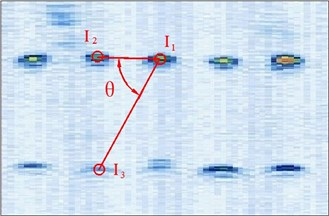a)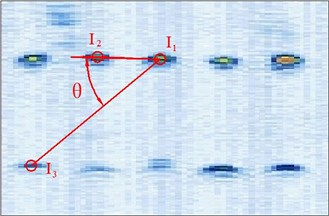b)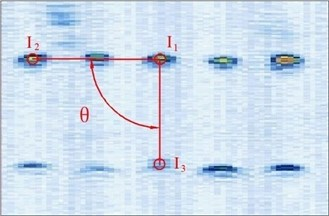c)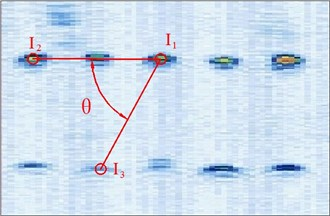d)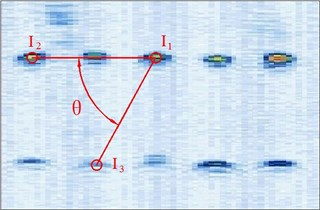e)

So in this article, the calibration reproducibility is the method to evaluate the calibration precision. The points ${P}_{i}$ were marked at the four corners of the image. The calibration reproducibility is in a matrix . The major factors causes the variations of the elements of calibration are: 1) Positioning errors when the stylus being placed into the hole on the phantom during phantom calibration (stylus measurements); 2) Placement incorrect when the phantom scanned by the probe freehand (phantom imaging or probe alignment); 3) Manual errors of the pixel coordinates of the crosses in the US image (manual image marking or segmentation). In the past researches, the third aspect is the most difficult to decease the errors. In our experiment, except for the method of homologous points in Figure 5, we select the points with different distances and angles to the reference point to calculate the matrix and average them as the final calibration matrix.

Table 1Precisions statistics of the calibration matrix elements

 Matrix elements Repeated phantom imaging (10 times) Repeated manual image marking (10 times) Repeated stylus measurements (10 times) Mean SD Range Mean SD Range Mean SD Range ${P}_{11}$ –0.0849 0.0031 0.0099 –0.0816 0.0011 0.0033 –0.0820 0.0005 0.0015 ${P}_{21}$ 0.9950 0.0026 0.0086 0.9960 0.0005 0.0019 0.9964 0.0008 0.0028 ${P}_{31}$ 0.0120 0.0034 0.0108 0.0099 0.0014 0.0042 0.0093 0.0010 0.0031 ${P}_{12}$ –0.9761 0.0011 0.0039 –0.9778 0.0005 0.0018 –0.9780 0.0005 0.0017 ${P}_{22}$ –0.0817 0.0035 0.0115 –0.0809 0.0106 0.0321 –0.0812 0.0103 0.0308 ${P}_{32}$ 0.1809 0.0025 0.0076 0.1811 0.0007 0.0026 0.1814 0.0016 0.0058 ${P}_{14}$ –87.7168 0.7141 2.6299 –87.3378 0.0961 0.2899 –87.3512 0.3594 1.0592 ${P}_{24}$ –22.3517 0.5400 1.9903 –22.5452 0.0458 0.1366 –22.5046 0.2651 0.7973 ${P}_{34}$ 22.7148 0.6171 1.8696 23.0869 0.0445 0.1187 22.7159 0.2343 0.6815

Table 2Reconstruction precisions of pixels along the center and edge of the US image

 Point depth(pixel) Repeated phantom imaging (10 times) Repeated manual image marking (10 times) Repeated stylus measurements (10 times) Center Edge Center Edge Center Edge 30 0.68 0.79 0.08 0.10 0.06 0.09 60 0.74 0.95 0.19 0.20 0.17 0.20 90 0.80 0.89 0.24 0.27 0.20 0.23 120 0.88 1.07 0.32 0.40 0.28 0.33 150 0.93 1.24 0.41 0.51 0.35 0.38 180 0.97 1.33 0.53 0.60 0.42 0.49 210 1.05 1.51 0.64 0.79 0.58 0.61 240 1.18 1.94 0.76 0.94 0.68 0.79 270 1.47 2.31 0.88 1.15 0.75 0.89 300 1.79 2.79 0.99 1.32 0.87 1.14

Repeat each of above three operations 10 times and computing variations of the calibration matrix. The probe was moved away from the strings and repositioned for each of the 4 images. This method ensures the independency of each image collection and presents the actual precision of the repeated calibration. The results are shown in Table 1. The first six rows are the rotation matrix elements and the last three rows are the translation vector in millimeters. The third column of calibration matrix is not shown, because it is the cross-product of the first two columns. The maximum discrete degrees are from 1.8696 mm to 2.6299 mm, it is show that the alignment of the probe procedure is the largest influence for the calibration precision. This is mainly caused by the ultrasound width. The calibration precision shows the same as other published methods reviewed by Hsu in 2009.

Points every 30 pixels were selected along the center and edge of the US images. Then translate these points in each image into the 3-D coordinate system, and then calculate the $\mathrm{\Delta }{P}_{CR}$, evaluating the point reconstruction accuracy. Repeat 10 times in phantom imaging, manual image marking and stylus measurements. The root mean square (rms) variation, the vector sum of the standard deviations SDs in the $x$, $y$ and $z$ direction, in the reconstructed pixel locations is a measure of the repeatability of the calibration method. The results are shown in Table 2. The calibration variability caused by image marking and stylus measurement was less than that caused by probe alignment. The calibration variability was higher when the depth is larger.

### 3.2. Reconstruction accuracy

One popular method for reconstruction accuracy is point reconstruction accuracy, which develops with the increased use of the stylus. Scan a point firstly, then calculate its 3D reconstructed location based on the calculation results. The 3D location of the point can be tested through the stylus, and the operation like in the Figure 3. This method was used transforming the points into the world coordinate system by Blackall in 2000, Muratore and Galloway Jr. in 2001, Pagoulatos in 2001 and into the sensor coordinate system by Lindseth in 2003. The discrepancy between the reconstructed coordinate and the stylus reading of the point reflects the reconstruction accuracy, i.e.:

5
$\mathrm{\Delta }{P}_{PA}=\left|{T}_{t←r}{T}_{r←p}{P}_{p}-{T}_{t←r}{T}_{r←i}s{P}_{i}\right|.$

This measurement includes errors from every component of the whole system. The thick beam width is the main causes for the error of the scan plane manual misalignment when using the point phantom. And segmentation error and position-sensor error are also the sources of error. So the point should be scanned from different positions and at different locations in the B-scan. A large number of images should be captured and the results averaged . The other, the same phantom and the same algorithm are not very appropriate to used assessing the point reconstruction accuracy, because the phantom errors or the algorithm errors will cause an offset in the calibration. These offset errors are easy to be neglected.

The other popular method for reconstruction accuracy is distance reconstruction accuracy, which is used before the stylus was developed. Many groups through measuring the distances between two points in 3D space and compare them with the distances in the phantom to obtain the reconstruction accuracy, such as Leotta in 1997, Prager in 1998; Blackall in 2000, Boctor in 2003, Lindseth in 2003, Leotta in 2004, Dandekar in 2005, Hsu in 2006, Krupa 2006. When using this method, we should note that the line image will be a distorted curve for line image calibrations, and the distance between the two end-points will be incorrect. So in this research we rotated the probe in different directions when scanned the phantom. The discrepancy between the reconstructed distance and the phantom distance of the two points reflects the distance accuracy:

6
$\mathrm{\Delta }{P}_{DA}=\left|\left|{P}_{p}^{1}-{P}_{p}^{2}\right|-\left|{T}_{t←r}{T}_{r←i}s{P}_{i}^{1}-{T}_{t←r}{T}_{r←i}s{P}_{i}^{2}\right|\right|.$

Therefore, the repeated precision reflects the stability degree of the calibration matrix, but does not provide an estimate of the validity of the calibration matrix, the effect of its 3-D transformation, so the point reconstruction accuracy and the distance reconstruction accuracy evaluation are necessary. The method is: rotate the probe in different directions when scanned the phantom. Apply the calibration matrix to translate the three point, ${I}_{1}$, ${I}_{2}$ and ${I}_{3}$, calculate the distance between ${I}_{1}$ and ${I}_{2}$, ${I}_{1}$ and ${I}_{3}$, then compare the distances with the real distances in the phantom. These data reflect the relative reconstruction accuracy. The experiment was divided into two groups, C: repeat the above to two procedures using the center 2 points to calculate the reconstruction accuracy, A: repeat the above to two procedures using the middle 4 points to calculate the reconstruction accuracy and B: repeat the above to two procedures using the edge 4 points to calculate the reconstruction accuracy. The results as seen in Table 3 show that the reconstruction accuracy of the center points is similar to that of the edge points. The point reconstruction accuracy is under ±0.15 mm.

Table 3Comparison reconstruction results

 Calibration source Point target variability rms (mm) Two points distance error (mean ± SD) C 0.89 0.08±0.02 A 0.94 0.09±0.02 B 1.12 0.15±0.06 C1 0.84 0.07±0.03 C2 0.91 0.05±0.02 A1 0.95 0.11±0.03 A2 0.94 0.10±0.03 A3 0.97 0.11±0.05 A4 0.91 0.08±0.01 B1 1.16 0.15±0.06 B2 1.03 0.09±0.04 B3 1.01 0.14±0.05 B4 1.15 0.13±0.05 A: 6 Center points reconstruction accuracyB: 4 edge points reconstruction accuracy

## 4. Conclusions

This paper presents a different image calibration method for freehand 3-D Ultrasonic testing system including cross-string phantom and corresponding calibration algorithm.

1) The phantom was designed in a simple construction. The ten crosses in the scanning plane provided the coordinates and characteristics points for the calibration algorithm, and the crosses and the strings out of the scanning plane guided the probe to align with the projects plane quickly and accurately.

2) Based on the ten crosses coordinates, the space vectors and the angle between the two vectors were calculated, furthermore the homologous points in the US image and in the phantom were obtained, matching them through the least-squares fitting method to calculate the spatial transformation matrix between the US image and the tracking sensor attached to the US probe.

3) The scanning results show that the scanning plane positioning time is no more than 5 s, faster than other method. And the precision and accuracy results demonstrate that the algorithm calculates the same accurate calibration matrix as that is obtained through the past reported methods.

31 October 2013
Accepted
05 December 2013
Published
30 June 2014
Keywords
ultrasound vibration
phased array
ultrasonic testing
image calibration
homologous points matching
Acknowledgements

This work was supported by: National Natural Science Foundation of China (61303098), Key Projects of Beijing Science & Technology Committee (H060720050330), 12-5 Connotation Construction Project for Shanghai University (B-8932-12-0131).